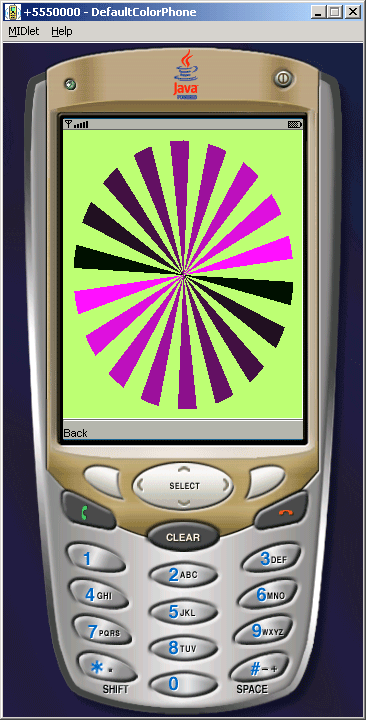Tutorials

# J2ME Canvas Example

This example illustrates how to create a game using GameCanvas class. In this example we are extending GameCanvas class to draw the circle and rotate the circle continuously.

This example illustrates how to create a game using GameCanvas class. In this example we are extending GameCanvas class to draw the circle and rotate the circle continuously.

# J2ME Canvas Example

A J2ME Game Canvas Example

This example illustrates how to create a game using GameCanvas class. In this example we are extending GameCanvas class to draw the circle and rotate the circle continuously. The GameCanvas class has following methods:

• flushGraphics():- This is the void type method, it flushes to display on the off-screen buffer.
• flushGraphics(int x, int y, int width, int height):- This is the void type method, it flushes to display of specified region on the off-screen buffer.
• getGraphics():- This is used to get the graphics objects.
• getKeyStates():- This is the integer type variable, it is used to find the states of the key.
• paint(Graphics g):- This is also the void type method, it is used to paint the canvas.

Other commands, input event, etc  methods inherited from Canvas class. The Canvas class has following methods:

• getGameAction(int keyCode)
• getHeight()
• getKeyCode(int gameAction)
• getKeyName(int keyCode)
• getWidth()
• hasPointerEvents()
• hasPointerMotionEvents()
• hasRepeatEvents()
• hideNotify()
• isDoubleBuffered()
• keyPressed(int keyCode)
• keyReleased(int keyCode)
• keyRepeated(int keyCode)
• paint(Graphics g)
• pointerDragged(int x, int y)
• pointerPressed(int x, int y)
• pointerReleased(int x, int y)
• repaint()
• repaint(int x, int y, int width, int height)
• serviceRepaints()
• showNotify()Source Code of CanvasGame.java

 ``` import javax.microedition.lcdui.*; import javax.microedition.lcdui.game.*; import javax.microedition.midlet.*; public class CanvasGame extends MIDlet{   private Command back;   private Display display;   final SweepGame game = new SweepGame();   public void startApp() {   back = new Command("Back", Command.BACK, 0);   game.start();   game.addCommand(back);   game.setCommandListener(new CommandListener(){   public void commandAction(Command c, Displayable s) {   game.stop();   notifyDestroyed();   }   });   display = Display.getDisplay(this);   display.setCurrent(game);   }   public void pauseApp() {}   public void destroyApp(boolean unconditional) {} } class SweepGame extends GameCanvas implements Runnable {   private boolean move;   private int radius;   private int diameter;   private int interval;   public SweepGame() {   super(true);   radius = 0;   diameter = 10;   interval = 0;   }   public void start() {   move = true;   Thread t = new Thread(this);   t.start();   }   public void stop() {   move = false;   }   public void render(Graphics g) {   int width = getWidth();   int height = getHeight();   g.setColor(183,251,121);   g.fillRect(0, 0, width - 1, height - 1);   int x = diameter;   int y = diameter;   int w = width - diameter * 2;   int h = height - diameter * 2;   for (int i = 0; i < 17; i=i+2) {   g.setColor(((17 - i) * 15 - 7),20,((17 - i) * 15 - 7));   g.fillArc(x, y, w, h, radius + i * 10, 10);   g.fillArc(x, y, w, h, (radius + 180) % 360 + i * 10, 10);   }   }   public void run() {   Graphics g = getGraphics();   while (move) {   radius = (radius + 1) % 360;   render(g);   flushGraphics();   try {   Thread.sleep(interval);   }   catch (InterruptedException ie) {}   }   } }```